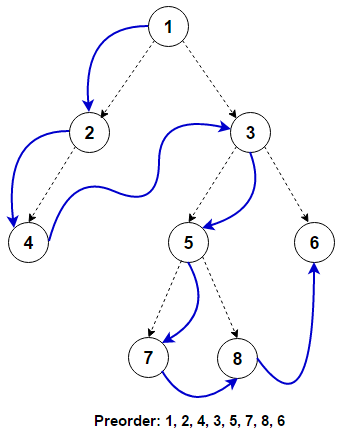# Preorder Tree Traversal | Iterative & Recursive

Given a binary tree, write iterative and recursive solution to traverse the tree using pre-order traversal in C++ and Java.

Unlike linked lists, one-dimensional arrays and other linear data structures, which are traversed in linear order, trees may be traversed in multiple ways in depth-first order (pre-order, in-order, and post-order) or breadth-first order (level order traversal). Beyond these basic traversals, various more complex or hybrid schemes are possible, such as depth-limited searches like iterative deepening depth-first search. In this post, preorder tree traversal is discussed in detail.

Traversing a tree involves iterating over all nodes in some manner. As tree is not a linear data structure, from a given node there can be more than one possible next node, so some nodes must be deferred i.e stored in some way for later visiting. The traversal can be done iteratively where the deferred nodes are stored in the stack or it can be done by recursion where the deferred nodes are stored implicitly in the call stack.

For traversing a (non-empty) binary tree in pre-order fashion, we must do these three things for every node N starting from root node of the tree:

(N) Process N itself

(L) Recursively traverse its left subtree. When this step is finished we are back at N again.

(R) Recursively traverse its right subtree. When this step is finished we are back at N again.

In normal pre-order traversal we visit left subtree before the right subtree. If we visit right subtree before visiting the left subtree, it is referred as reverse pre-order traversal.As we can see only after processing any node, the left subtree is processed, followed by the right subtree. These operations can be defined recursively for each node. The recursive implementation is referred to as depth-first search (DFS), as the search tree is deepened as much as possible on each child before going to the next sibling.

## Java

#### Iterative implementation –

To convert above recursive procedure to iterative one, we need an explicit stack. Below is a simple stack based iterative algorithm to do pre-order traversal.

iterativePreorder(node)

if (node = null)
return
s -> empty stack
s.push(node)
while (not s.isEmpty())
node -> s.pop()
visit(node)
if (node.right -> null)
s.push(node.right)
if (node.left -> null)
s.push(node.left)

## Java

Above solution can be further optimized by pushing only the right children to the stack.

## Java

The time complexity of above solutions is O(n) and space complexity of the program is O(n) as space required is proportional to the height of the tree which can be equal to number of nodes in the tree in worst case for skewed trees.

References: https://en.wikipedia.org/wiki/Tree_traversal(1 votes, average: 5.00 out of 5)Loading...

Please use our online compiler to pre code in comments.
Like us? Please spread the word and help us grow. Happy coding 🙂